×

Search anything:

# Improve your Python code speed

#### Python Software EngineeringGet this book -> Problems on Array: For Interviews and Competitive Programming

Python is one of those languages that provide a multitude of ways to do the same thing. This flexibility comes at a cost, the choices we make impact the prefomance of our code. Good programs strive to achieve balance between memory and time resources.

The impact might seem trivial for small programs but the impact becomes so evident when code complexity grows. In the receding paragraphs, I will point out ways to improve your python code.

## Choose the right data structure

You might be tempted to type in browser omnibox something like this "best python data structure". But right is different from best and not to mention, such a query is likely to bring up results pertaining to religious wars.

When choosing a data structure, you might consider the following:

1. How often are you going to search the data structure
2. Memory used by the data structure, Do you plan to use less memory?
3. Do you allow duplicates in the data structure?

The essence of all this is to assess your situation and try to take the optimal decision.

## String Concatenation

Due to the immutability of strings, string concatenation with a plus (+) has to create a new block of memory and then copy both strings into the new memory location.

``````import timeit

str_1 = "A"*1000
str_2 = "B"*2000

def concat_with_plus(str_1, str_2):
return str_1 + str_2

def concat_with_format(str_1, str_2):
return "%s%s".format(str_1, str_2)

print("Concatenation with plus takes ", timeit.timeit("concat_with_plus(str_1, str_2)", "from  __main__ import concat_with_plus, str_1, str_2"), "seconds")
``````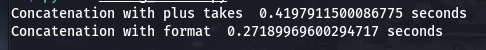Concatenation with format() function takes almost half of the time it takes for the plus concatentation function.

So instead of using plus(+), you can swap it with f strings, format().

## Use xrange instead of range

For those using python 3.x, this is not of concern as the range() function works like xrange() under the hood.

The concern here is about memory, xrange() lazily loads values in the range provided to it whereas range() immediately allocates memory for all the values in the range provided.

``````import sys

def test_with_range_func():
return range(1, 10000)

def test_with_xrange_func():
return xrange(1, 10000)

print "range() has used ", sys.getsizeof(test_with_range_func()), "bytes"
print "xrange() has used ", sys.getsizeof(test_with_xrange_func()), "bytes"
``````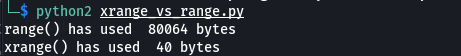## Use built-in functions

We tend to expend time in optimizing our code but we can hardly beat the efficiency of built in functions.

Before you write that custom function, be sure that there is no built-in function that does the same thing you are trying to accomplish.

``````import timeit

numbers = range(1, 1000)

def custom_sum():
total = 0
for number in numbers:
total += number

def built_in_sum():
total = sum(numbers)

print("Custom sum() function takes ", timeit.timeit("custom_sum()", "from __main__ import custom_sum, numbers"), "seconds.")
print("Built In sum() function takes ", timeit.timeit("built_in_sum()", "from __main__ import built_in_sum, numbers"), "seconds.")
``````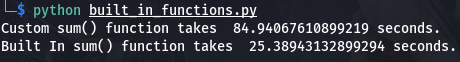Its worth noting the custom sum() function takes more than a minute and the builtin function sum() takes less than half a minute.

## Use list comprehensions

``````import timeit

def get_even_numbers_less_100():
even_numbers = []
for number in range(1, 100):
if number%2 == 0:
even_numbers.append(number)
return even_numbers

def get_even_numbers_less_100_lc():
even_numbers = [number for number in range(1, 100) if number%2 == 0]
return even_numbers

print("List comprehension takes ", timeit.timeit("get_even_numbers_less_100_lc()", "from __main__ import get_even_numbers_less_100_lc"), "seconds.")
print("List construction with append and conditionals takes ", timeit.timeit("get_even_numbers_less_100()", "from __main__ import get_even_numbers_less_100"), "seconds.")
``````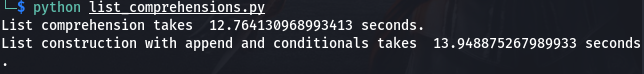The explanantion behind the margin lies in the bytecode generated by each of the functions.

List comprehension bytecode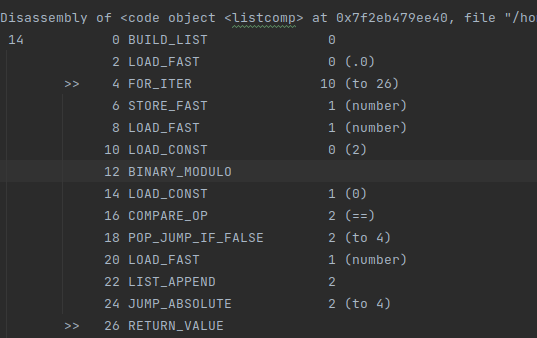Instead of the LOAD_METHOD opcode, python knows that its dealing with list comprehension and thus uses the LIST_APPEND opcode (offset 22).

For loop bytecode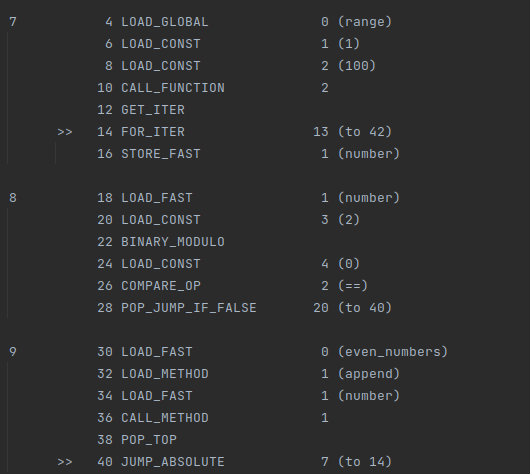I have only excerpted the bytecode for the loop- starts at line 7.
In each iteration, the append() method is loaded (offset 32), translated and called. Thus, the append() loading time in each iteration adds up to contribute to the delay.

## Keep up to date with latest python releases

There is a diverse community of developers behind python and with each new release, new features might be introduced, existing ones might be improved.

Endeavour to acquaintancize with new python releases and probably upgrade your code to the latest releases.

Perhaps we have already talked about generators in regard to the usage of xrange() function. You can also write your own generators in cases where xrange() or range() is not appropriate.

``````def fib(limit):

# Initialize first two Fibonacci Numbers
a, b = 0, 1

# One by one yield next Fibonacci Number
while a < limit:
yield a
a, b = b, a + b

for fib_no in fib(5):
print(fib_no, end=', ')
``````

This is a fibonacci sequence generator, Instead of a return statement, I use a yield statement.
To make a generator function, you use a yield statement instead of return.
And this function can be used like the range() or xrange() functions.

## Avoid global variables

Besides being a sign of a broken window, retrieving global variables is slower than retrieving local variables.

``````i = 0
def func():
global i # Avoid this
``````

## Use Cython

The Cython language is a superset of the Python language that additionally supports calling C functions and declaring C types on variables and class attributes. This allows the compiler to generate very efficient C code from Cython code.
This implies python can benefit from fast execution nature of C.

Cython adds speed to python while keeping the simplicity and beginner freindliness intact.

Cython Factorial

``````cpdef int factorial(int x):
cdef int y = 1
cdef int i
for i in range(1, x+1):
y *= i
return y
``````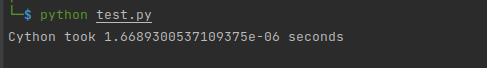Pythoh Factorial

``````def factorial(x):
y = 1
for i in range(1, x+1):
y *= i
return y
``````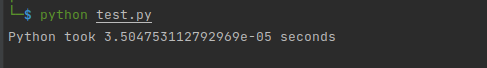Cython Code syntax and running cython code is beyond the scope of this article. Please refer to the cython documentation: https://docs.cython.org/

Cython is not meant to replace python but rather to improve python speed, use cython when doing heavy mathematical operations and loop iterations.

## Use in membership operator

This comes back to using the built-in features. Avoid using loops when checking for membership of a value in an iterable. Use the membership in operator instead.

``````token = 4
numbers = range(1, 100, 3)

# Using the in operator
if token in numbers:
pass

# Using the for loop to check membership
for num in numbers:
if token == num:
pass
``````

## Use profiling tools

Profiling tools come in handy when trying to single out parts of code that need to be optimized. A profile is a set of statistics that describes how often and for how long various parts of the program executed.

Python includes two profilers:

1. cProfile
2. profile

Please refer to the profiler documentation: https://docs.python.org/3/library/profile.html

## Use concurrency

Concurrency is having two events occuring at the same time. Python's concurrency slightly obscures the above statement due to its single threaded natures imposed by the Global Interpreter Lock (GIL).
Python interpreter runs on a single CPU and thus python multithreading implies a thread time shared execution i.e. Threads take turns in execution on a single CPU.

We can distribute the workload via multiple threads in order to complete the task at hand faster.

Threading, asyncio, and multiprocessing libraries can be used to achieve concurrency in python.

multiprocessing is different from the other two, it creates a new instance of the python interpreter and uses that to run part of your program. Multiprocessing implements true parallelism because its able to utilize the more than one CPU in a multi cored CPU system. However this comes at a cost.

Use concurrency for only CPU bound and I/O bound problems

Usage of python concurrency libraries is beyond the scope of this article, I urge to look at the individual library documentations.#### Kirabo Ibrahim

BSTE Student in Computer Science at Makerere University, Uganda.